# How to Use the VLOOKUP Formula in Functions in Excel 2007 and 2010

Robbie mostly writes about Skyrim but also occasionally sheds light on the oddities of Microsoft applications such as Excel and Outlook.

Both Excel 2007 and 2010 have an enormous number of functions available. Today I will be looking at one of the most powerful Excel functions, VLOOKUP, and how it can be used in formulas. What VLOOKUP does in essence is that it looks for a value within a table of data and returns some specific information related to that value. To illustrate how to harness the power of VLOOKUP, we will be looking at some examples:

• Firstly, we will be using VLOOKUP to fetch price information on products from our pricing catalogue so that we can determine the value of an order.
• Secondly, VLOOKUP will be used to complete a user’s name and address in the order by looking up the customer’s customer ID in our customer database and returning their details.
• Lastly, we will look at using VLOOKUP to look up data in another workbook.

## Examples of How to Use VLOOKUP in Excel 2007 and 2010

Suppose you own a music shop/website. You have a list of orders from customers in your order book, your catalogue containing all your prices, and a database of your customer’s details.

Each customer, once they have made a purchase, is assigned a customer number and their details are entered into the database. When an order comes in, you record that order in the order book using the customer number into your spreadsheet.

In our first example, we want Excel to be able to tell you the value of a customer order. You know that the customer wants the Green album from R.E.M. on CD for example. Using VLOOKUP, Excel 2007 orl 2010 compares your order with your catalogue and comes back with the price.

In our second example, we want Excel to fetch a customer’s address automatically when we enter their customer ID into an order.

Let’s look at each example now in more detail, before also investigating how to use VLOOKUP to fetch data from another workbook.

## How to Use VLOOKUP to Fetch Pricing Information for Products in Excel 2007 and 2010

In our first example, we are going to use VLOOKUP to fetch the price of our products (each different media has a different cost). In my example below, I have the pricing details on the right and the order details on the left. If my music shop was an actual shop, chances are that the data would be on different sheets in the same workbook or even another workbook entirely but I have them next to each other to make it easier to see how the formulas fit together.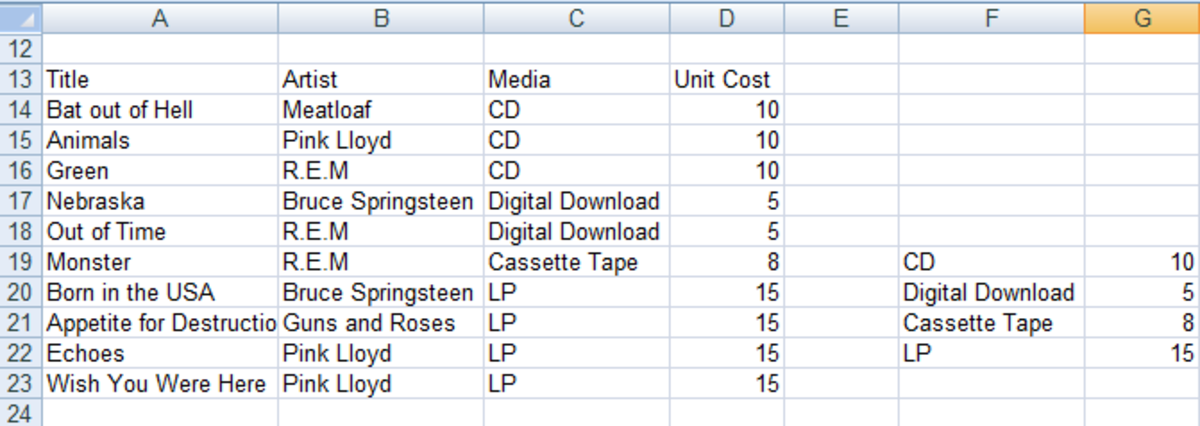Order book and Pricing catalogue used by VLOOKUP to calculate order value in Excel 2007 and Excel 2010.http://robbiecwilson.hubpages.com/

The next step is the formula itself. I will show first the finished formula and then explain each part.

=VLOOKUP(C14,\$F\$19:\$G\$22,2,FALSE)

The formula is made up of four parts:

• The first part tells Excel what I am asking VLOOKUP to look for (in this example, I am looking for CD so I give Excel the cell C14 as a reference).
• The second part defines the range of cells (which in this case is \$F\$19:\$G\$22) I am asking Excel to search for the contents of cell C14 (CD).

Note: The \$ next to the column and row of the cell, tells Excel that they do not change from one formula to the next, they are always constant. This ensures that Excel always uses your exact range for the VLOOKUP formula when you copy it to other cells in your workbook.

• The third part is a little complicated, so let’s break the formula down to show exactly what Excel does and how it uses the third part of the formula:
Scroll to Continue
1. We have asked VLOOKUP to find the contents of cell C14 (part 1) in the range \$F\$19:\$G\$22 (part 2).
2. Excel looks in the first column of the range (\$F19-\$F22) and finds CD in cell F19.
3. Part 3 tells Excel to look in the second row when it finds CD so when it finds CD in F19, it returns the value of 10 (G19) which is in the second row and is exactly what we wanted it to do!
• The final part lets Excel know whether you are looking for an exact match (False), or an approximate match (True). As I am looking for an exact match, in this case I chose False.

Note: This part can be complicated and particularly when using True, Excel can on occasion return incorrect results. I have an article that shows how to use the true and false value correctly so that VLOOKUP returns a correct result every time. That article can be found here.

In the picture below, you can see the full formula as well as all the cells it references.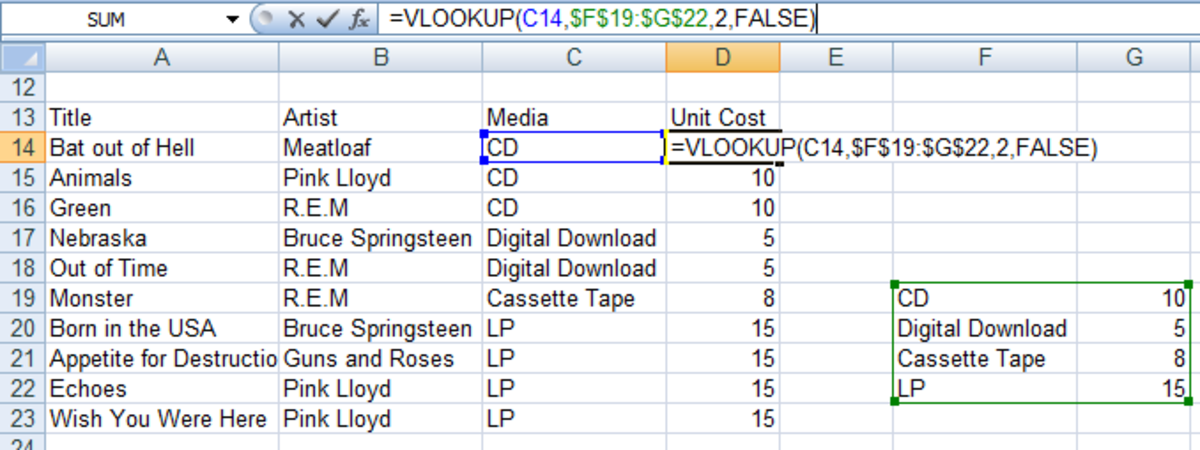VLOOKUP formula in Excel 2007 and Excel 2010 looking up media pricing in a Pricing catalogue.http://robbiecwilson.hubpages.com/

## Using VLOOKUP to Complete the Address of Customers in an Order Using Their Customer Number in Excel 2007 and 2010

For our next example, each time a customer orders something from my shop, I enter their customer number in their order and would like Excel to auto-populate their names and addresses using VLOOKUP. I have all these details stored in my customer database and would like Excel to fetch these for me automatically. I have the data set up as below with my order book on the left and the customer database on the right.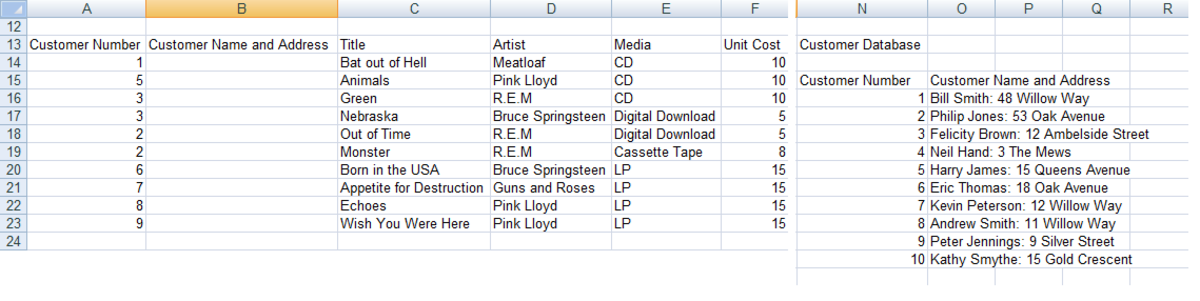Order book and Customer database used by VLOOKUP to fetch customer's details in Excel 2007 and Excel 2010.http://robbiecwilson.hubpages.com/

The formula is set up in exactly the same way as the example above.

=VLOOKUP(A14,\$N\$16:\$O\$25,2,FALSE)

Once again, the four steps are the same as the previous example.

• 1 (contained in cell A14) is what you are asking Excel 2007 or 2010 to look for.
• \$N\$16:\$O\$25 is the range of cells that you want Excel to look in.
• 2 is the row that contains the data you want Excel to return.
• FALSE is tells Excel to return data only if it finds an exact match.

The figure below shows the formula and the cells it references so you can see VLOOKUP in action.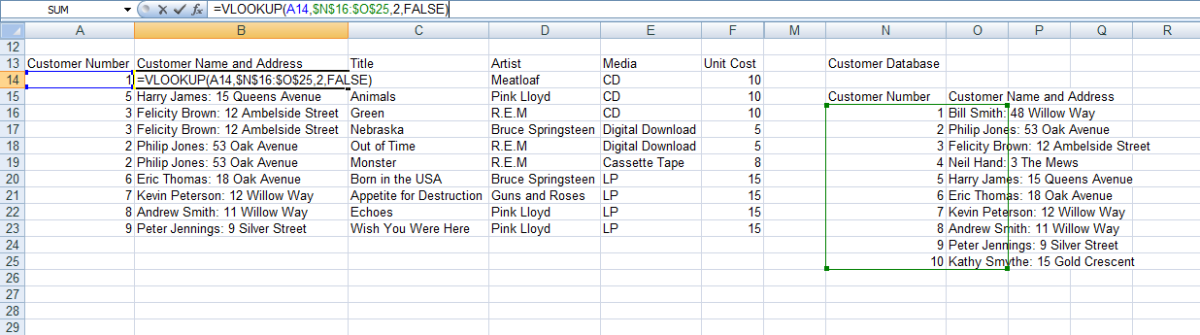VLOOKUP formula in Excel 2007 and Excel 2010 fetching customer's details from the Customer database.http://robbiecwilson.hubpages.com/

## Using the VLOOKUP Function in a Formula When Your Data Is in Another Excel 2007 or 2010 Workbook

If some of your data that VLOOKUP will refer to is in another workbook (say for example, my customer database might be maintained by a different member of my team and entered into another workbook), it is quite straightforward to add this range to a new or existing formula.

=VLOOKUP(E14,’C:\[Book1]Sheet1’!\$A\$1:\$B\$4,2,FALSE)

The format is:

• Firstly, the location of the file (in my case C:\) with a ’ in front as shown above
• The name of the file in [] (in my case Book2.xlsx).
• The Sheet that the data is on (in my case Sheet 1) followed by a ’!.
• And finally the range of data as normal.

There is another way of doing it which many will find easier as you don’t have to amend any formulas.

Open both Excel 2007 workbooks and click on the Restore Window button (as shown below) for the documents rather than Excel itself so that the workbook only takes up part of the overall Excel window.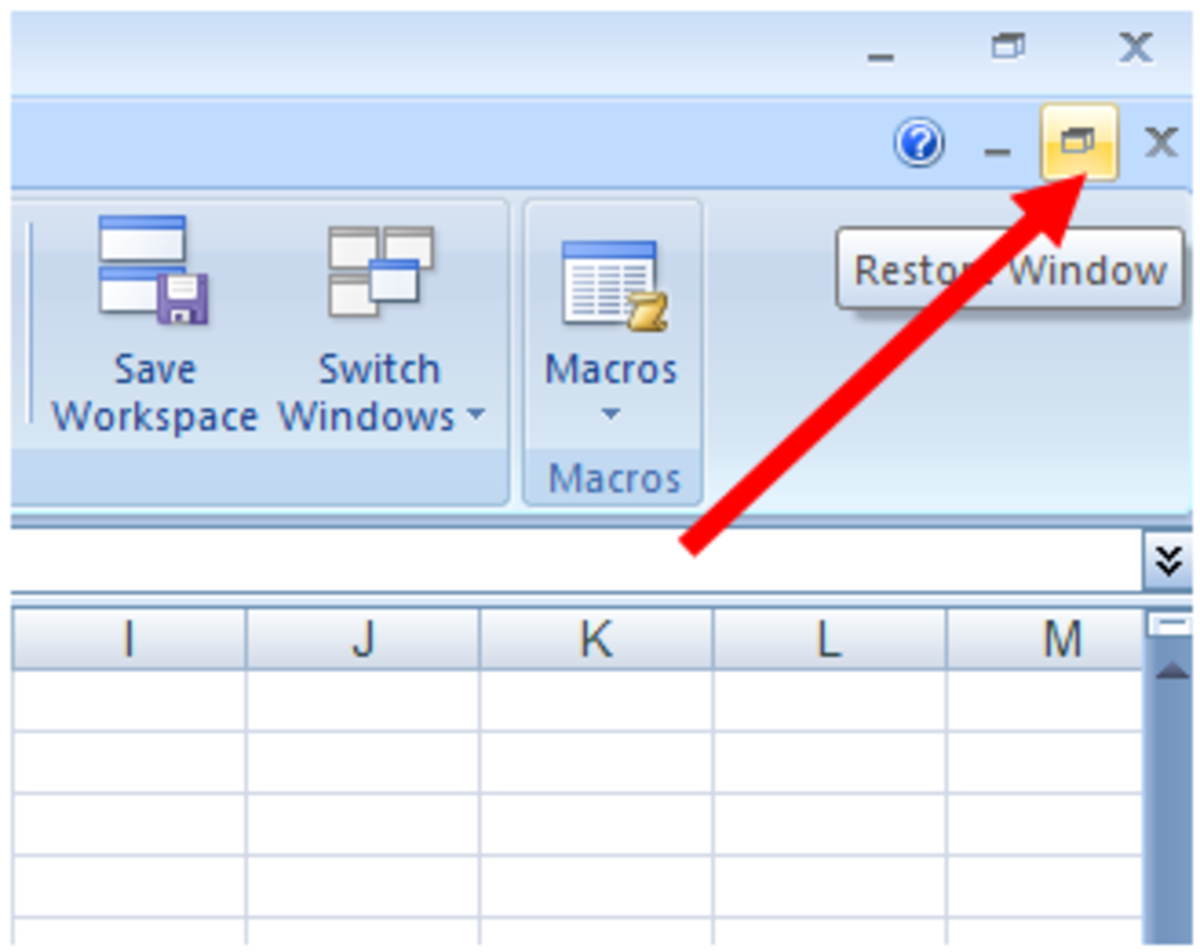How to stop a workbook from taking the entire Excel 2007 or Excel 2010 window to allow two workbooks to be viewed at once.http://robbiecwilson.hubpages.com/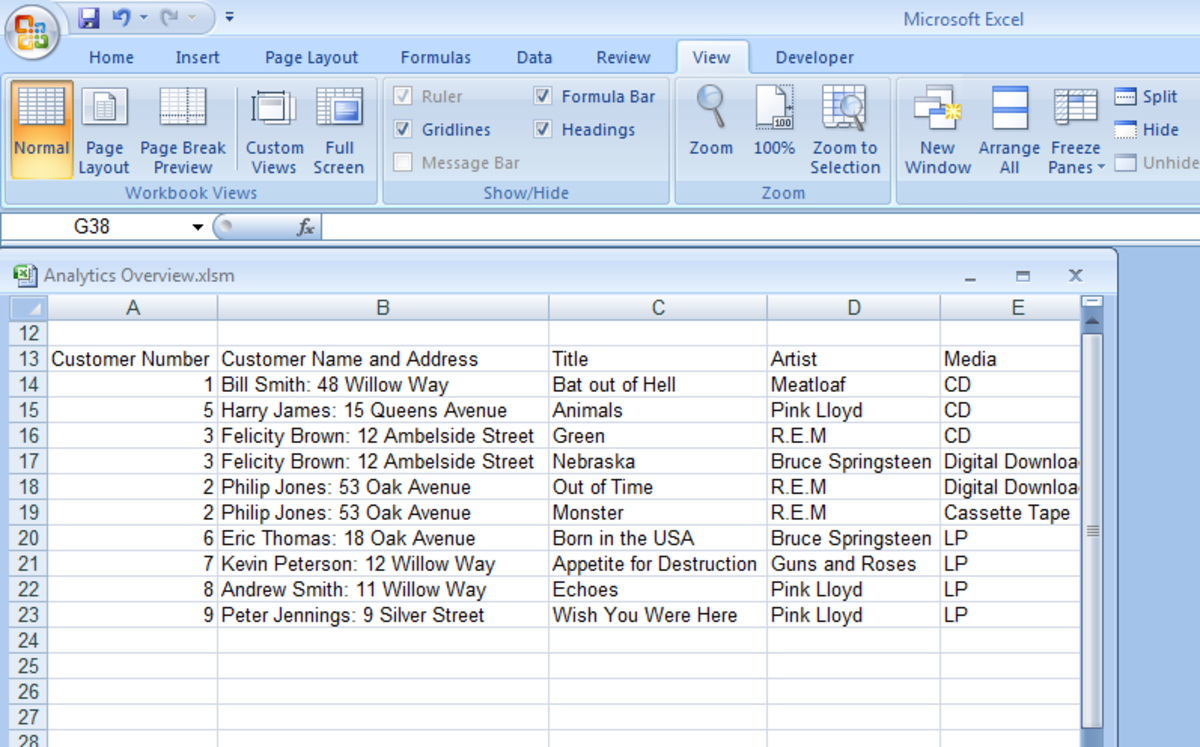Showing a windowed Excel workbook allowing another workbook to be viewed at the same time in Excel 2007 and Excel 2010.http://robbiecwilson.hubpages.com/

Open the second workbook and position it so that it is next to the other workbook you have open.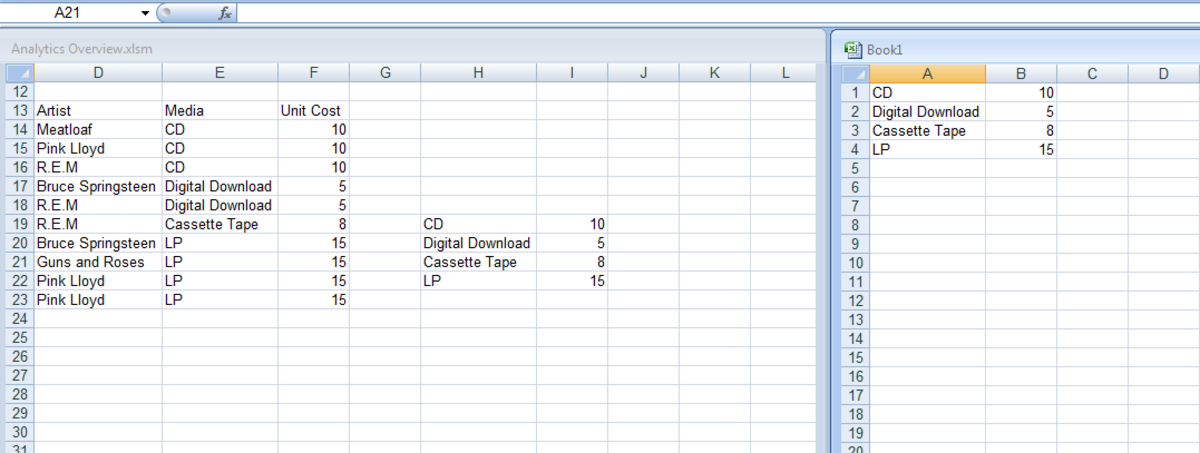Two workbooks side by side in Excel 2007 and Excel 2010.http://robbiecwilson.hubpages.com/

Enter in the first part of the formula as normal and then when selecting the range for the second part, select the data in the second workbook as shown below.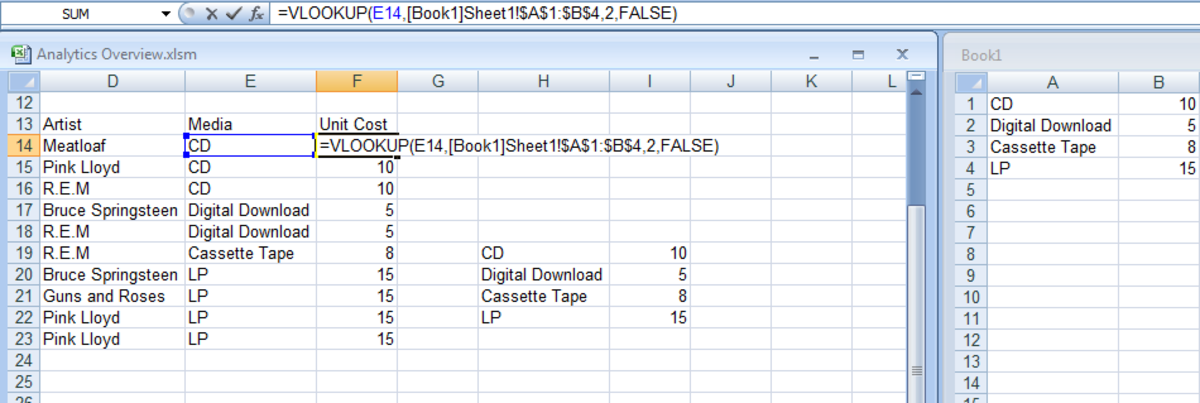Illustrating a VLOOKUP formula using two workbooks in Excel 2007 and Excel 2010.http://robbiecwilson.hubpages.com/

You will notice that the path doesn’t look quite right in this formula with both workbooks open.

=VLOOKUP(E14,[Book1.xlsx]Sheet1!\$A\$1:\$B\$4,2, FALSE)

As soon as you close the workbook containing the range (in my case Book 1), the formula changes to something that looks more logical.

=VLOOKUP(E14,'C:\[Book1.xlsx]Sheet1'!\$A\$1:\$B\$4,2,FALSE)

## Excel 2007 and 2010 Treats Your Data as a Table When You Use VLOOKUP

A great feature of Excel 2007 and 2010 and also the VLOOKUP function is that when you use VLOOKUP, Excel converts your data into a table. It still looks and feels like normal data, but when I for example put a new order into my workbook in row 24 and enter in the customer number of the person who has placed a new order into cell A24, Excel automatically fills in the VLOOKUP data (in my case it enters in Harry James: 15 Queens Avenue into B24 next to the five I entered into cell A24). Once I enter what it is that the customer ordered, Excel will then auto-fill the Unit Cost and enter it into cell F24 for me.

Should you want to break this behaviour, leave a blank row and then carry on entering in new data and Excel will not auto-fill cells using VLOOKUP (if I enter 7 into cell A26, Excel will not auto-fill as A25 is empty so it knows that the table has been completed).

## And now a quiz to see what we've learned today!

For each question, choose the best answer. The answer key is below.

1. How many parts are there in a VLOOKUP formula?
• 2
• 4
• 3
2. What does a \$ in front of a cell reference tell Excel?
• That the cell references don't change when copied
• That the cell contains currency information
• That the cell contains a formula
3. What does the fourth part of a VLOOKUP formula do?
• Checks if the formula will return a numerical answer
• Check if the formula contains an error
• Tell Excel whether to look for an exact or an approximate match

1. 4
2. That the cell references don't change when copied
3. Tell Excel whether to look for an exact or an approximate match

If you got 1 correct answer: Well done, almost there!

If you got 2 correct answers: You passed, nice one!

If you got 3 correct answers: Well done, top of the class, give yourself a virtual pat on the back!

## Conclusion

VLOOKUP is a very powerful formula that can dramatically reduce the amount of data entry and the errors that are associated with it, by looking up and fetching data for you automatically. By working through the VLOOKUP function in two examples step by step, I hope that I have succeeded in making this daunting looking function accessible to you and that you will now be able to use it in your own spreadsheets and that it also saves you a lot of time and typing!